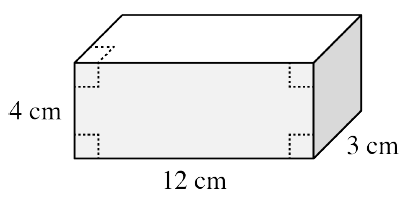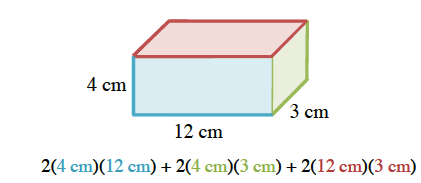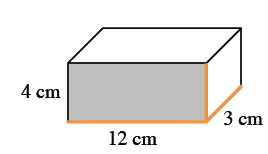### Home > CC2MN > Chapter 10 > Lesson 10.3.2 > Problem10-78

10-78.Use the rectangular prism shown at right to complete parts (a) through (d) below.

1. Calculate the surface area of the prism. Show all of your subproblems.

To find the surface area, find the area of each face of the prism.

There are $6$ faces in a rectangular prism.
Each of the three different faces shown in the image have an opposite face, so find the area of each of those $3$ faces and multiply it by $2$.2. Explain why the exponent for area units is $2$ (that is, cm2).

How many terms do you multiply together to find area?

To find area, you multiply two dimensions.
Just as $(x)(x)=x^2,(\text{cm})(\text{cm})=\text{cm}^2$.

3. Calculate the volume of the prism.

To find the volume of a rectangular prism, multiply the base, height, and width together.

$\text{Volume}=(12\ \text{cm})(4\ \text{cm})(3\ \text{cm})$4. Explain why the exponent for volume units is a $3$ (that is, $\text{cm}^{3}$).

See part (b).